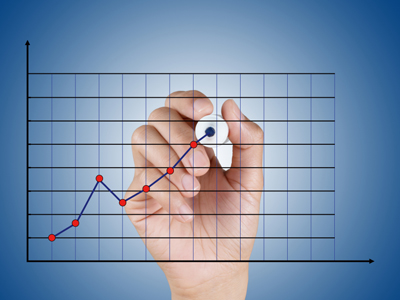In graphs, the key variable is always plotted on the x axis.

# Unit 4 - Key/Independent Variable

Variables are an important part of any experiment. Control variables are ones which remain the same throughout and dependent variables are the ones being measured. In this GCSE Biology quiz we look at independent or key variables - the ones which are subject to change.

The key independent variable is the one variable that you change in your investigation or experiment and it is the basis of your hypothesis. All other variables in your investigation should be kept the same, after all, if you changed two variables, how would you know which one has had the effect you observed? The dependent variable is the variable you hope will be changed as you alter the values of the key independent variable. It is the one that you will be measuring.

When you write out your hypothesis, most people will naturally place the key independent variable first. For example, "I think that as the light intensity is increased, the seedlings will grow taller because ...". It could have also been written as I think that the seedlings will grow taller as the light intensity increases because ..." so it doesn't happen all the time. When deciding on the key independent variable, the most reliable way of working out what it is, is by checking your hypothesis for the variable that you think will cause something else to change in a predictable way. In our example, you can see that the light intensity is the key independent variable because it is the one that you think will cause the seedlings to grow taller.

As with all variables, the data can be continuous or discrete. Continuous data can have any value and so you must decide on the range and number of readings that you will take. You must consider how many readings you think are practical, for instance, if you have temperature as the key independent variable, in a school laboratory, -5oC would be the lowest practical temperature you could achieve (using a mixture of water, ice and salt) and would you really need to take readings at 1oC intervals? Of course not! Every 5 or 10 degrees would give you sufficient results from which to draw a conclusion. You also need to take into account safety factors, so using concentrated hydrochloric acid would not be possible as it is not allowed to be used in GCSE science experiments because it is dangerously corrosive. You are more limited in your choices if your key independent variable has discrete values, but you will probably still need to make decisions based on practicality and safety.

1.
The key variable is...
the variable that is changed by the experimenter
the variable that is controlled by the environment of the experiment
the variable that is measured by the experimenter
the variable that is always decreased in value
During the experiment, you can add extra values for the independent variable if you need to as it is in your control. This can help to make your results more accurate and reliable
2.
In graphs, the key variable is always plotted on the...
x axis
y axis
both axes
does not matter
The key variable is always plotted on the x axis and the dependent variable is always plotted on the y axis
3.
The key variable is the factor we are...
changing
keeping constant
ignoring
measuring
You decide the value of the key variable when you design the experiment. It can also be referred to as the independent variable or the key independent variable
4.
Controlled variables are always kept...
the same
different
larger
smaller
Controlled variables are all the other variables, except the key variable and the dependent variable
5.
I think that as we increase the temperature, the number of germinating seeds will increase. The dependent variable is ...
temperature
number of seeds
increase
decrease
What you intend to measure is the dependent variable
6.
You decide these values at the planning stage of your investigation.
Dependent variable only
Control variables only
Dependent and control variables
Key independent variable and control variables
It is up to you to fix their values
7.
A variable in an experiment is...
a factor we can change or measure or control
a factor in the environment
something which changes
something which stays the same
There may be factors outside of your control. These variables need to be monitored so that you can decide if they had an effect on your results during the experiment
8.
Uncontrolled variables may make the results...
unreal
accurate
unreliable
true
Careful control of the variables is the key to a successful investigation
9.
I think that as the mass increases, the speed will decrease. Which is the key variable?
Mass
Speed
Increase
Decrease
The key (independent) variable is the one that you think will cause something else to change
10.
If we change the value of a factor affecting the experiment, it must be the ...
key variable
a controlled variable
dependent variable
an anomaly
During the experiment, this must be the only variable you change in order to ensure a fair test